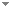## Works by Alain Louveau

9 found
Order:
1. Borel Equivalence Relations Induced by Actions of the Symmetric Group.Greg Hjorth, Alexander S. Kechris & Alain Louveau - 1998 - Annals of Pure and Applied Logic 92 (1):63-112.
We consider Borel equivalence relations E induced by actions of the infinite symmetric group, or equivalently the isomorphism relation on classes of countable models of bounded Scott rank. We relate the descriptive complexity of the equivalence relation to the nature of its complete invariants. A typical theorem is that E is potentially Π03 iff the invariants are countable sets of reals, it is potentially Π04 iff the invariants are countable sets of countable sets of reals, and so on. The proofs (...)

Export citationBookmark11 citations
2. Analytic Ideals and Cofinal Types.Alain Louveau & Boban Velickovi - 1999 - Annals of Pure and Applied Logic 99 (1-3):171-195.

Export citationBookmark9 citations
3. On the Quasi-Ordering of Borel Linear Orders Under Embeddability.Alain Louveau & Jean Saint-Raymond - 1990 - Journal of Symbolic Logic 55 (2):537-560.
We provide partial answers to the following problem: Is the class of Borel linear orders well-quasi-ordered under embeddability? We show that it is indeed the case for those Borel orders which are embeddable in R ω , with the lexicographic ordering. For Borel orders embeddable in R 2 , our proof works in ZFC, but it uses projective determinacy for Borel orders embeddable in some $\mathbf{R}^n, n , and hyperprojective determinacy for the general case. Direct download (8 more) Export citationBookmark3 citations 4. Two Results on Borel Orders.Alain Louveau - 1989 - Journal of Symbolic Logic 54 (3):865-874. We prove two results about the embeddability relation between Borel linear orders: For$\eta$a countable ordinal, let$2^\eta$(resp.$2^{<\eta}$) be the set of sequences of zeros and ones of length$\eta$(resp.$<\eta$), equipped with the lexicographic ordering. Given a Borel linear order$X$and a countable ordinal$\xi$, we prove the following two facts. (a) Either$X$can be embedded (in a$\triangle^1_1(X,\xi)$way) in$2^{\omega\xi}$, or$2^{\omega\xi + 1}$continuously embeds in$X$. (b) Either$X\$ can (...)

Export citationBookmark3 citations

Export citationBookmarkExport citationBookmarkExport citationBookmark8. Recursivity and Capacity Theory.Alain Louveau - 1985 - In Anil Nerode & Richard A. Shore (eds.), Recursion Theory. American Mathematical Society. pp. 285--301.
Export citationBookmarkExport citationBookmark